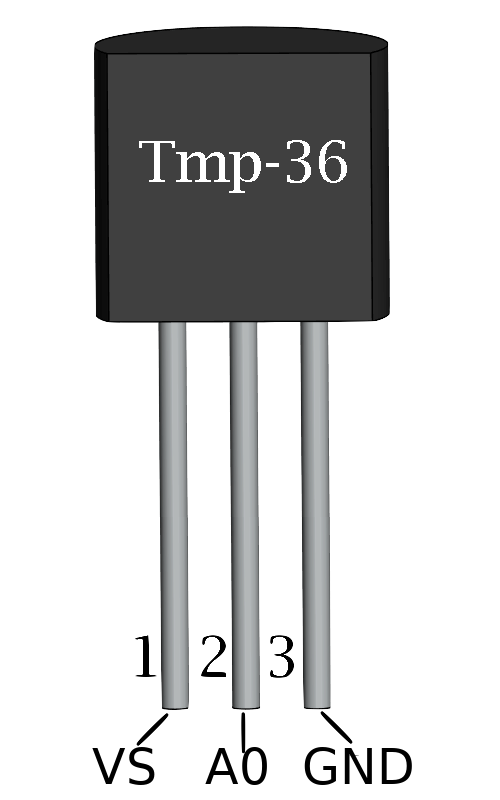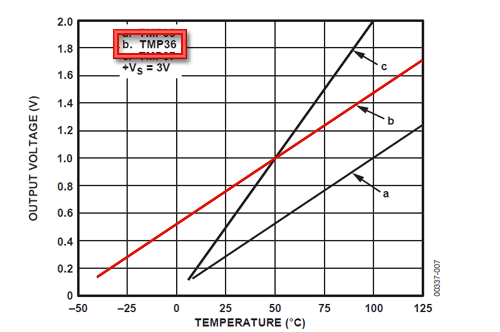# # Analog Pins

Analog pins are labeled on the Arduino Uno from A0 to A5. The Arduino Uno board has an analog to digital converter. The analog signal input from the analog pin will go through the converter. The converter has a 10-bit resolution which will return an integer from 0 to 1023.

We can read from an analog pin by using

``````analogRead(pinNumber);
``````

The Arduino platform already defines the PINs with `A0`, `A1` ... So we can use that directly in our code.

``````analogRead(A0);
``````

## # TMP36 Temperature Sensor

TMP36 is an analog temperature sensor. That means the input to the Arduino from the sensor is an analog signal. The sensor has 3 pins. Input voltage, ground and output voltage. We connect the input voltage to the 5V output from the Uno board, the ground to GND pin of the Uno and the output voltage to the A0 pin.From the documentation, we can learn what the voltage will be based on the temperature. The following graph represents the voltage from the sensor relative to the temperature.First, we need to read the value from the analog pin

``````int sensorInput = analogRead(A0);
``````

The analog value we read is passed by the converter, which returns a value from 0 to 1023. From this value we can find the percentage of the input reading. We divide the reading by 1024.

We know that the voltage can maximum be 5V and minimum 0V. As the source of voltage is coming from the 5V pin on our Uno.

To find the voltage from the sensor, we multiply the percentage of the reading by 5.

``````//find percentage of input reading
double temp = (double)sensorInput / 1024;
//multiply by 5V to get voltage
temp = temp * 5;
``````

Based on the documentation (and from the chart), we can convert the voltage to temperature value.

``````temp = temp - 0.5;    //Subtract the offset
temp = temp * 100;    //Convert to degrees
``````20